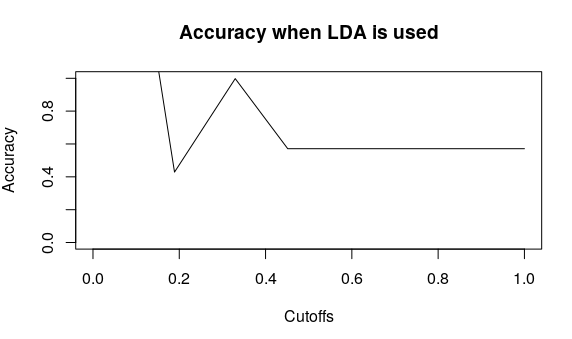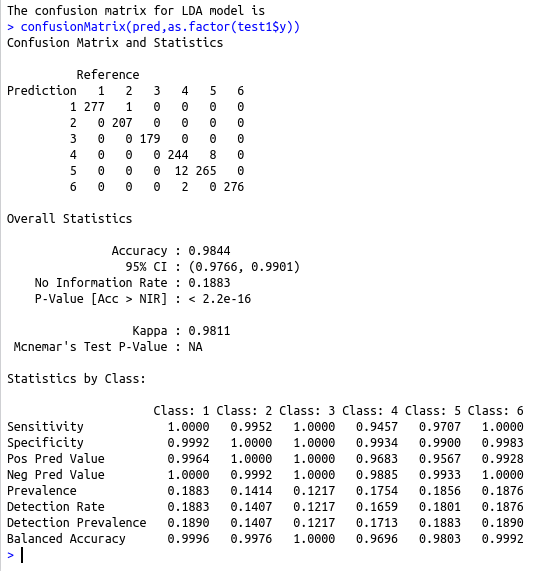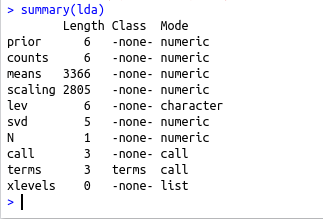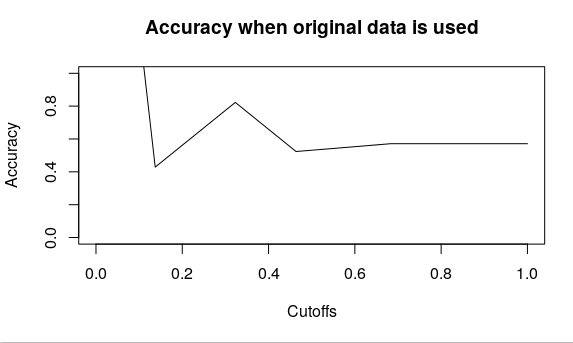• #5, First Floor, 4th Street Dr. Subbarayan Nagar Kodambakkam, Chennai-600 024 Landmark : Samiyar Madam
• pro@slogix.in
• +91- 81240 01111

### How to reduce the dimension of a given data set using Linear Discriminant Analysis and build a machine learning model?

###### Description

To reduce the dimension of a given data set using linear discriminant analysis and build a machine learning model

#### Functions used :

lda(formula,data) – To compute the Linear discriminant analysis

#### Data set :

Human activity recognition using Smart phone

#### Libraries required :

require(MASS)
library(caret)

library(naivebayes)

library(AUC)

###### Process

Load the required libraries

Load the data set

Split the data frame for train and test

Compute the Linear Discriminant Analysis using the train data

Predict using the test data

Compute the confusion Matrix

Build the naive bayes model using train data

Predict using the test data

Compute the confusion matrix

Compare the confusion matrix obtained from the two model and interpret the result

###### Sapmle Code

#load the required libraries
require(MASS)
library(caret)
library(naivebayes)
library(AUC)
#Load the data set
#To Split 80% of data as training data
smp_size train_ind train1 test1 #Perform linear discriminant analysis
lda #Take the summary
summary(lda)
pred1=predict(lda,test1)
pred=pred1\$class
cat(“\nThe confusion matrix for LDA model is \n”)
confusionMatrix(pred,as.factor(test1\$y))
#Build the naive bayes model using the original train data
nb1 #Predict using the original test data
pred2=predict(nb1,test1)
#Compute the confusion matrix
cat(“\nThe confusion matrix for Naive bayes model is \n”)
print(confusionMatrix(pred2,test1\$y))
#To interpret the result
plot(accuracy(pred,test1\$y), type = “l”,main=”Accuracy when LDA is used”)
plot(accuracy(pred2,test1\$y), type = “l”,main=”Accuracy when original data is used”)
plot(roc(pred,test1\$y), type = “l”,main=”ROC when LDA is used”)
plot(roc(pred2,test1\$y), type = “l”,main=”ROC when Original data is used”)

###### Screenshots